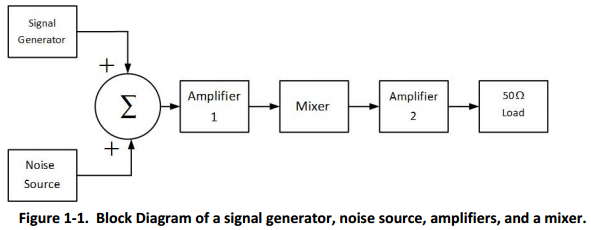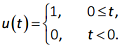### What is the rms voltage at the output of amplifier

Assignment Help Electrical Engineering
##### Reference no: EM131500058

Q1. As shown in figure 1-1, the output of a noise source and the output of a signal generator are summed together. The resulting signal plus noise is the input of a system. The system consists of the cascade combination of:

Amplifier 1, a mixer (two port frequency converter), and amplifier 2. The block diagram of this system is shown in figure 1-1.The signal is a bandpass modulated signal with a 1 MHz bandwidth.

The centre frequency of the signal is 900 MHz.

Amplifier 1 and amplifier 2 have bandpass transfer function characteristics. Amplifier 1 has a centre frequency of 900 MHz. The mixer down-converts a 900 MHz signal to 100 MHz. Amplifier 2 has a centre frequency of 100 MHz. Both amplifier 1 and amplifier 2 have noise equivalent bandwidths of 10 MHz. The amplified and frequency converted signal does not have any signal distortion at the 50 Ω load resistor.

The noise source has a noise temperature 2,000 Kelvin.

The three devices are specified by:

amplifier 1,         Noise Figure=1.4 dB,          Gain=15 dB,

mixer,                Noise Figure= 13 dB,          Gain= 6 dB,

amplifier 2,         Noise Figure=16.0 dB,         Gain=60 dB.

It is required to have a signal to noise ratio, (Ps/Pn)dB = 16 dB, at the 50 Ω load resistor at  the output of amplifier 2, where  Ps is the signal power and Pn is the noise  power.

Part A -

To achieve this, what is the required RMS (root mean square) signal level at the signal generator output in volts? Note, the output of the signal generator is measured across a 50 Ω resistive impedance.

Part B -

Corresponding to the signal generator output that achieves (Ps/Pn)dB = 16 dB, what is the rms voltage at the output of amplifier 2 in volts?

Q2. A bandpass radio signal s(t) has complex envelope representation,

s(t) = Re[s~(t)ej2πf_ct]

where,

s~(t) = Aee-at u(t)The signal is put through a bandpass filter with impulse response h(t), where,

h(t) = Re[h~(t)ej2πf_ct]

where h~(t) = Cee-βt u(t)

What is the output of the filter, y(t) as a function of t , A, C, α, β, θ and Φ?

Hint: y(t) = ½Re[y~(t)ej2πf_ct], where y~(t) = s~(t)*h~(t) and * is the convolution operator.

Q3. A communication system uses QPSK modulation.

The bit error rate requirement for this system is PB = 10-6.

The transmission frequency is fo = 2.4 GHz.

The transmitter power is 1 Watt.

The feed line loss between the transmitter and the transmit antenna is 2 dB.

The transmit antenna is omnidirectional and has a gain of 0 dBi.

The transmit antenna height is 20 meters

For the receiver, the low noise amplifier has a noise figure of 7 dB, the feedline loss is 1.5 dB, the antenna temperature is 290 K, and the receive antenna gain is 3 dBi. The demodulator implementation loss is 1 dB.  The receive antenna height is 1.5 meters.

A. Assuming free space loss in the channel, plot the data rate that the link supports for distances between 100 meters and 20 km. Use a log-log graph to plot the data rate in bits/s as a function of distance, d, in meters.

B. Assuming a two-ray propagation loss model, plot the data rate that the link supports from dc to 20 km, where dc = 4hthr/λ = 4hthrfo/c.

On the same graph as part A, plot the data rate in bits/s as a function of distance, d, in meters.

Verified Expert

The work is about three questions in communication systems. Question 1 is about calculation of the RMS value based on various parameters given, this is calculated for the signal generation and output side Question 2 is about calculation of the relation between various parameters given in the question and this is accomplished using Laplace transform Question 3 is about calculation of the data rates and making plots based on distance variation

#### Design an operational amplifier circuit

Design an operational amplifier circuit to perform the function of the black box - Design a current-to-voltage converter circuit to achieve the required bipolar output voltage

#### Calculate dimension of the channel width for the drivers

Calculate the dimension of the channel width W for the drivers and respective dimensions of W and L for C and RL. To assist you with the calculation, you can select appropriat

#### Calculate the dimension of the channel width

Calculate the dimension of the channel width W for the drivers and respective dimensions of W and L for C and RL. To assist you with the calculation, you can select appropriat

#### Maximum demand project assignment

Show worst case volt drops diagrammatically. Make statement regarding compliance - Show Prospective Fault Current levels in Main & all Sub-mains based on your selected Transf

#### How the fluid level could be digitally controlled

Consult any classical control text1 to obtain a block diagram of an analog fluid control system. Modify the block diagram to show how the fluid level could be digitally cont

#### Describe the data presented to the operator

State the minimum number of printed-circuit cards required to construct a basic controller, naming each of the cards and produce a sketch to show the construction of the data

#### How the conversion process modifies the signal

The purpose of this experiment is to observe how the conversion process modifies the signal. A circuit board having separate sample-and-hold (S/H), A/D and D/A sections is p

#### Compare unshielded twisted pair and coaxial cabling types

Compare cat. 6 unshielded twisted pair, coaxial, and single mode fiber optic cabling types. Describe one or two of the advantages and disadvantages associated with using eac

### Reviews

#### inf1500058

6/14/2017 5:56:04 AM

Expertsmind.com is really the best assignment help site, ever. The experts here are so helpful. I had such a variety of questions about my graduate paper and the expert truly helped me. Furthermore I got refinement on my paper.

#### len1500058

5/18/2017 2:52:22 AM

Your Assignment must be submitted on the ELEC426 iLearn page. The report should be submitted in the PDF format. The following file naming format should be used: FamilyName_GivenName_Assignment.pdf. Late submissions will incur a penalty of 10% for each working day or part of beyond the submission deadline. A completed and signed cover sheet must be submitted with the report. A scanned version of the completed cover sheet should be attached in front of the report (forming a single pdf document).

### Write a Review